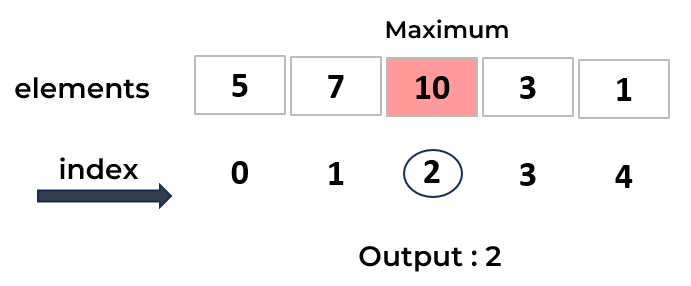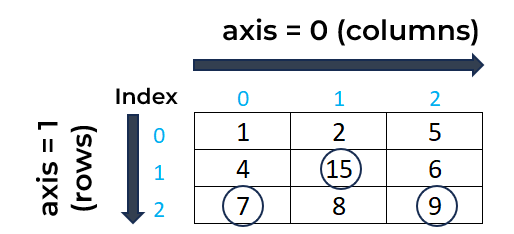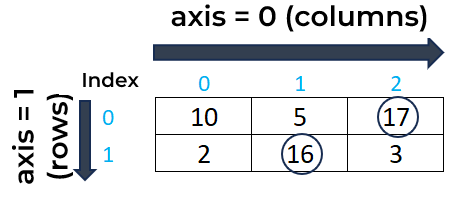# NumPy argmax() Function : Learn with Examples

In this tutorial, we will see how to use the NumPy argmax() function in Python along with examples.The numpy.argmax() function in Python is used to find the indices of the maximum element in an array.

## Syntax of NumPy argmax() Function

Below is the syntax of the NumPy argmax() function:

```import numpy as np
np.argmax(array, axis, out)
```
• array : NumPy array.
• axis : (optional). Specify the axis to find the indices of the maximum value in two dimensional arrays.
• out : (optional). It is used to store the output of the function.
Example 1 : argmax() Function in One-Dimensional Array

The following code uses the argmax() function from the NumPy package to return the index of the largest value in the one-dimensional array named "my_array".In this array, the maximum value is 10 which lies at the 2nd index. Keep in mind that indexing starts from 0 in python so the 2nd index refers to the third element in the array.

```import numpy as np
my_array = np.array([5,7,10,3,1])
np.argmax(my_array)
```
``````
# Output
2
```
```
Example 2 : argmax() Function in Two-Dimensional Array

In this example, we have created a sample 2D array. In this array, the largest value is 15 which lies at the 4nd index as indexing starts from 0 in python instead of 1.

```# 2D array
my_array = np.array([[1, 2, 5], [4, 15, 6], [7, 8, 9]])

# Index of the maximum element
np.argmax(my_array)
```
``````
# Output
4
```
```
Example 3 : Finding Maximum Element Indices per Column

When we use `axis=0` argument in the argmax() function, it means that we want to find the indices of the maximum elements along each column of the array. In simple words, it returns the index of the maximum value for each column.```# 2D array
my_array = np.array([[1, 2, 5], [4, 15, 6], [7, 8, 9]])

# Finding the indices of the maximum elements along each column (axis=0)
print(np.argmax(my_array, axis=0))
```
``````
# Output
[2 1 2]
```
```
Example 4 : Finding Maximum Element Indices per Row

When we use `axis=1` argument in the argmax() function, it means that we want to find the indices of the maximum elements along each row of the array. In simple words, it returns the index of the maximum value for each row.```# 2D array
my_array = np.array([[10,5,17],[2,16,3]])

# Finding the indices of the maximum elements along each row (axis=1)
print("Indices of maximum elements per row:", np.argmax(my_array, axis=1))
print("Indices of maximum elements per column:", np.argmax(my_array, axis=0))
```
``````
# Output
Indices of maximum elements per row: [2 1]
Indices of maximum elements per column: [0 1 0]
```
```
Example 5 : Store Result using out Parameter

We can use the `out` parameter in the argmax() function to store the result which is the index of the maximum element.

```my_array = np.array([5,7,10,3,1])

# Creating an output array to store the index of maximum element
out_array = np.array(0)
np.argmax(my_array,out=out_array)
print("Index of maximum element:",out_array)
```
``````
# Output
Index of maximum element: 2
```
```

In two-dimensional array, it is important to create an empty NumPy array with the number of columns in "my_array" and integer data type to store result.

```my_array = np.array([[7, 4, 6], [6, 5, 7], [7, 8, 9]])

# Creating an output array to store the indices of maximum elements along each column
out_array = np.empty(my_array.shape, dtype=int)

np.argmax(my_array, axis=0, out=out_array)
print("Indices of maximum elements:",out_array)
```
``````
# Output
Indices of maximum elements: [0 2 2]
```
```
Related Posts